# Normal Distribution Approximation of the Binomial Distribution

Author: Sophia Tutorial
##### Description:

Calculate the mean, standard deviation, or variance using the normal distribution approximation of a binomial distribution.

(more)

Sophia’s self-paced online courses are a great way to save time and money as you earn credits eligible for transfer to many different colleges and universities.*

No credit card required

37 Sophia partners guarantee credit transfer.

299 Institutions have accepted or given pre-approval for credit transfer.

* The American Council on Education's College Credit Recommendation Service (ACE Credit®) has evaluated and recommended college credit for 32 of Sophia’s online courses. Many different colleges and universities consider ACE CREDIT recommendations in determining the applicability to their course and degree programs.

Tutorial
what's covered
This tutorial will cover the normal distribution approximation of the binomial distribution. Our discussion breaks down as follows:

1. Binomial Distribution
2. Normal Distribution Approximation of the Binomial Distribution

## 1. Binomial Distribution

First, let's review the binomial distribution itself:Using that formula, you can create a probability distribution for all the values of k: zero successes, one success, two successes, all the way up to n successes.

 k P(X = k) 0 1 2 3 ... n

That can be made into a histogram, where the x-axis represents the values of k, the number of successes; and the y-axis is the relative frequency of those successes. Each bucket for the values of k go up to the height corresponding to the probability.

Just like all distributions, that histogram is going to have a mean and a standard deviation. The mean is fairly obvious to calculate.

EXAMPLE

Suppose you rolled a die six times. How many threes would you expect? What if you rolled it 60 times or 600 times? How many threes would you expect?

What you should be thinking is if you rolled it six times, you'd expect one of those six rolls to be a three. If you rolled it 60 times you'd expect about 10 threes. If you rolled it 600 times, you'd expect about 100 threes.

You were multiplying by 1/6 because 1/6 was the probability (1/6 of six is one). Therefore, 60 times 1/6, which is the probability of a three, gives you 10 as the expected value.

big idea
The average, or the expected value, is going to be the number of trials times the probability of success.

For a binomial distribution, we can identify the mean, standard deviation, and variance:

formula
Mean of a Binomial Distribution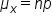Standard Deviation of a Binomial Distribution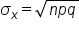Variance of a Binomial Distribution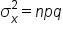## 2. Normal Distribution Approximation of the Binomial Distribution

Every distribution has three key features:

1. Center
3. Shape

You just dealt with center and spread by finding the mean and the standard deviation.

formula
Center of a Binomial DistributionBut what about the shape? Shape of this distribution is affected by two things: both n and p.

Look at the following distributions for a high, low, and middle probability. Notice how the distribution changes as the probability changes and number of trials change.

High Probability
p = 0.90
Number of Trials Distribution
10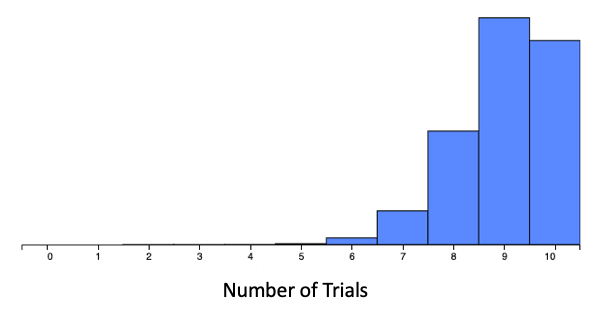100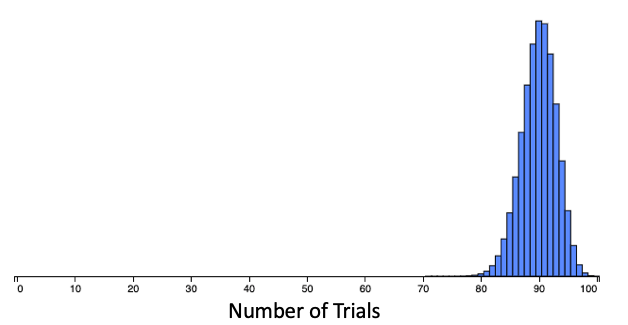When the probability of success is very high, the distribution is skewed very heavily to the left when the number of trials is low. But as the number of trials increases, the distribution is nearly symmetric. It's a little skewed to the left, but not heavily skewed as with the lower number of trials.

Low Probability
p = 0.15
Number of Trials Distribution
10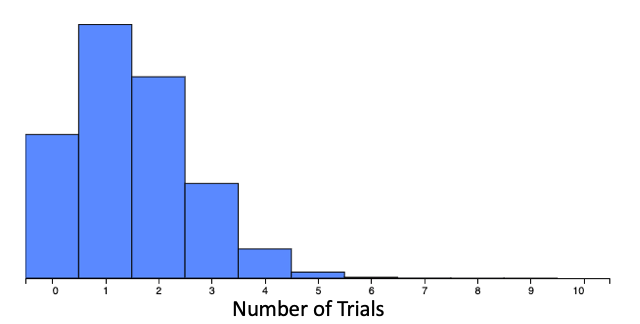100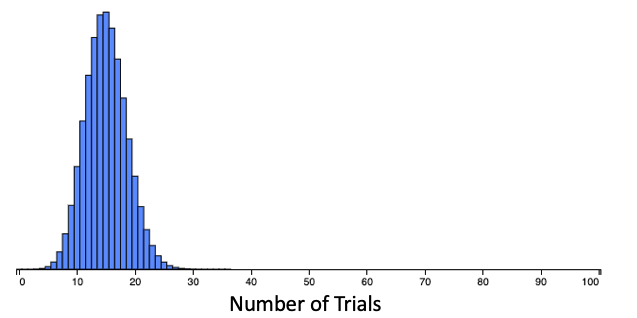When the probability of success is very low, the distribution is skewed very heavily to the right when the number of trials is low. But as the number of trials increases, the distribution is now only slightly skewed to the right.

High Probability
p = 0.50
Number of Trials Distribution
10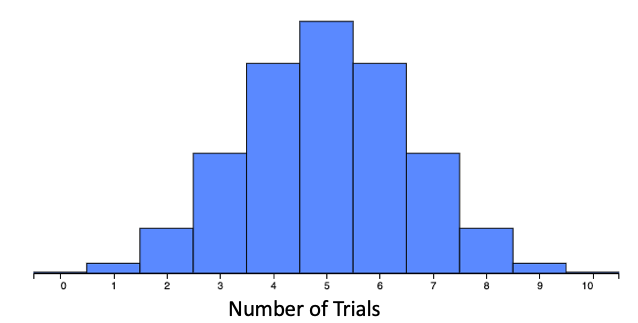100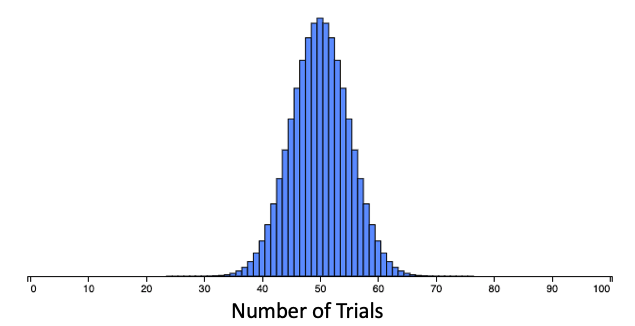When the probability of success is in the middle, near 0.50, the distribution becomes nearly symmetric when the number of trials is low. As the number of trials increases, the distribution stays symmetric.

That's what you should see when we look at the normal distribution approximation of the binomial distribution.

big idea
When n is high, the distribution is approximately normal. The only exceptions are when the value of p is very low or very high. When n is low, the skew, if any, is more prominent.

This is a critical concept. This means that when you have a large number of trials, the distribution of binomial probabilities is nearly normal, with the mean of what you found the mean to be, and standard deviation of what you found the standard deviation to be. Ultimately what you're finding is the binomial distribution with parameters n and p--which is what makes the binomial look like what it looks like--looks a lot like the normal distribution with that mean and that standard deviation.

The distribution has to be large enough to satisfy these two conditions:

1. The mean, np, has to be at least 10.
2. The expected number of failures, nq, has to be at least 10.

This means that you had to be far enough off of the left-hand side and far enough off the right-hand side. When you had that distribution, it looked normal when you were safely in the middle of the distribution, and not near the very ends. These two conditions have to be satisfied. This makes looking at a lot of these problems much easier.

EXAMPLE

Suppose a baseball player gets a hit 28% of the time when he comes to bat. What’s the probability that he gets over 30 hits in his next 95 at bats?

Using the old way, you would have to find the probability that he gets exactly 31 hits, plus the probability that he gets exactly 32, all the way up to the probability that he gets exactly 95 hits. But if you completed all 65 calculations, you would get a probability of 0.185, or 18.5%.The new way uses the normal approximation. First, check to see if the distribution is large enough to satisfy the two conditions above. We know there are 95 trials, the probability of success is 0.28, and the probability of failure is 1 minus 0.28, or 0.72.Both conditions are satisfied, np and nq are both bigger than 10. We can now find the mean and standard deviation.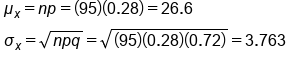Using the mean of 26.6 and a standard deviation of 3.763, the normal distribution, or the binomial distribution, is going to look very much like this and we can find the probability of hitting over 30 hits.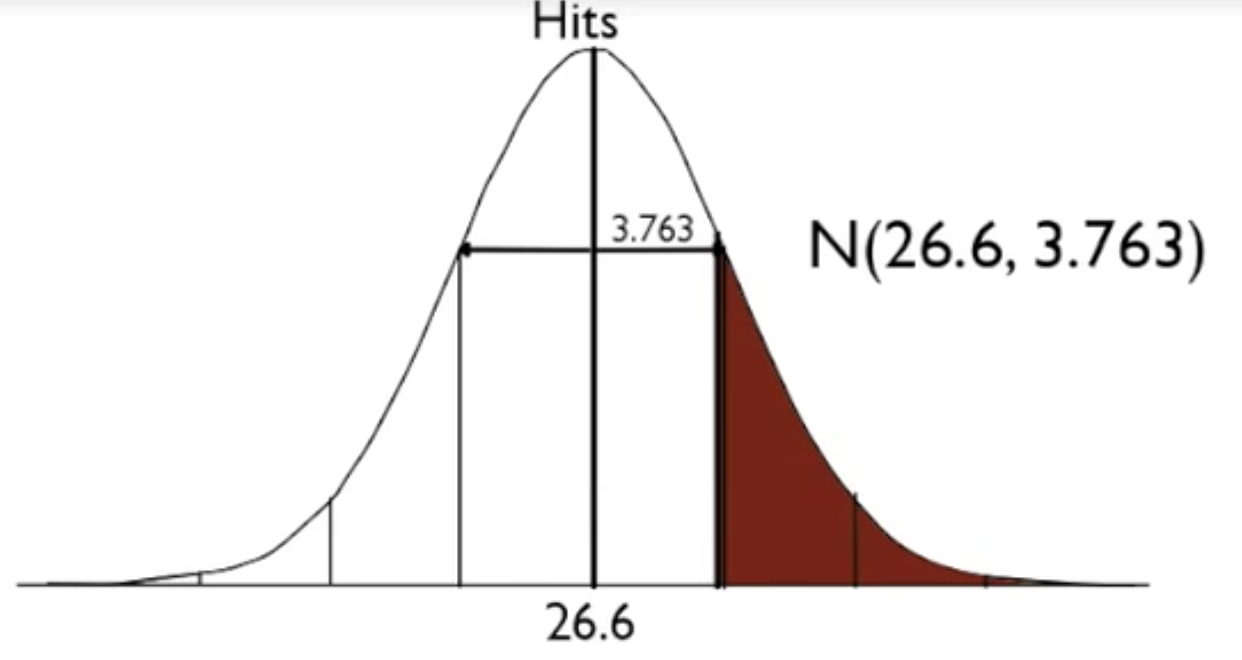Based on this normal distribution, calculate out a z-score and use a z-table to find the probability that way.This is almost the same as what you would get if you used the binomial calculations.
term to know

Normal Distribution Approximation of the Binomial Distribution
If a random variable has a binomial distribution, the number of trials is sufficiently large, and the probability of success is not too close to 0 or 1, the variable's distribution can be approximately modeled using a normal distribution.

summary
The normal distribution is a good approximation for the binomial under certain conditions: n has to be large, and p has to be not too extreme, meaning not too high and not too low. You can use the mean and standard deviation of the binomial as the mean and standard deviation for the normal, and use z-scores to find the probabilities. This simplifies the problem.

Good luck!

Source: Adapted from Sophia tutorial by Jonathan Osters.

Terms to Know
Normal Distribution Approximation of the Binomial Distribution

If a random variable has a binomial distribution, the number of trials is sufficiently large, and the probability of success is not too close to 0 or 1, the variable's distribution can be approximately modeled using a normal distribution.

Formulas to Know
Center of a Binomial DistributionMean of a Binomial Distribution